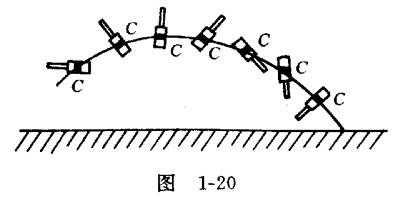# 重心与质心首先看重心，任何物体都可以看作是由很多微粒所组成，每个微粒都受到竖直向下的重力的作用，由于地球很大，这些力可认为彼此平行。因此，又可以说任何一个物体都受到很多的平行力——物体的各微粒所受的重力的作用。所有这些重力的合力就等于整个物体的重力，它可以根据平行力的合成法则来求得。这些平行力的合力作用点就叫做物体的重心（如图1－18的 C 点）。

${m_i}{{\bf{a}}_i} = {{\bf{F}}_i} + \sum\limits_j {{{\bf{f}}_{ij}}} \tag{1}\label{1}$

${m_i}{{\bf{a}}_i} = \sum\limits_i {{F_i}} + \sum\limits_i {\sum\limits_j {{f_{ij}}} } \tag{2}\label{2}$

$\sum\limits_i {{m_i}{{\bf{a}}_i} = {\bf{F}}} \tag{3}\label{3}$

$m{\bf{a}} = {\bf{F}}\tag{4}\label{4}$

（4）式中 m ＝ $$\sum\limits_i {{m_i}}$$，为物体的总质量。然而，现在我们研究的物体运动并非平动，故（3）式不能写成（4）式。不过，我们可换一种思想来考虑问题。

${{\bf{a}}_C} = \frac{{\bf{F}}}{m}\tag{5}\label{5}$

${{\bf{a}}_C} = \frac{{\sum\limits_i {{m_i}{{\bf{a}}_i}} }}{m}\tag{6}\label{6}$${{\bf{r}}_C} = \frac{{\sum\limits_i {{m_i}{{\bf{r}}_i}} }}{m}\tag{7}\label{7}$

$\begin{array}{l}{x_C} = \frac{{\sum\limits_i {{m_i}{x_i}} }}{m}\\{y_C} = \frac{{\sum\limits_i {{m_i}{y_i}} }}{m}\\{z_C} = \frac{{\sum\limits_i {{m_i}{z_i}} }}{m}\end{array}\tag{8}\label{8}$

${{\bf{a}}_C} = \frac{{{{\mathop{\rm d}\nolimits} ^2}{{\bf{r}}_C}}}{{{\mathop{\rm d}\nolimits} {t^2}}} = \frac{{\sum\limits_i {{m_i}{{\bf{a}}_i}} }}{m} = \frac{{\bf{F}}}{m}\tag{9}\label{9}$

（9）式就是（5）式或（6）式。所以，（7）式所表示的质心的确存在，它的加速度符合（5）式的要求。（9）式表明：对于物体（或质点组）有一个质量中心（质心），它的运动好像是一个质点的运动，这个质点的质量就等于物体的全部质量，受到的力就是物体所受到的所有外力的矢量和。可见，质心的运动与内力无关，若外力的矢量和等于零，则物体的质心静止或作匀速直线运动。质心除具有上述几个重要性质外还有很重要的性质。例如，若外力作用线不通过质心，则物体既作平动又作转动；若外力的作用线通过质心，则物体只作平动而不发生转动。又如，经计算可知，不管物体的形状如何，其重力势能总可以用其质心高度乘以物体的总质量和重力加速度来表示。

2006 - 2023，推荐分辨率1024*768以上，推荐浏览器Chrome、Edge等现代浏览器，截止2021年12月5日的访问次数：1872万9823 站长邮箱